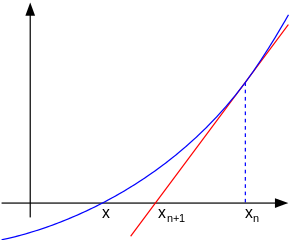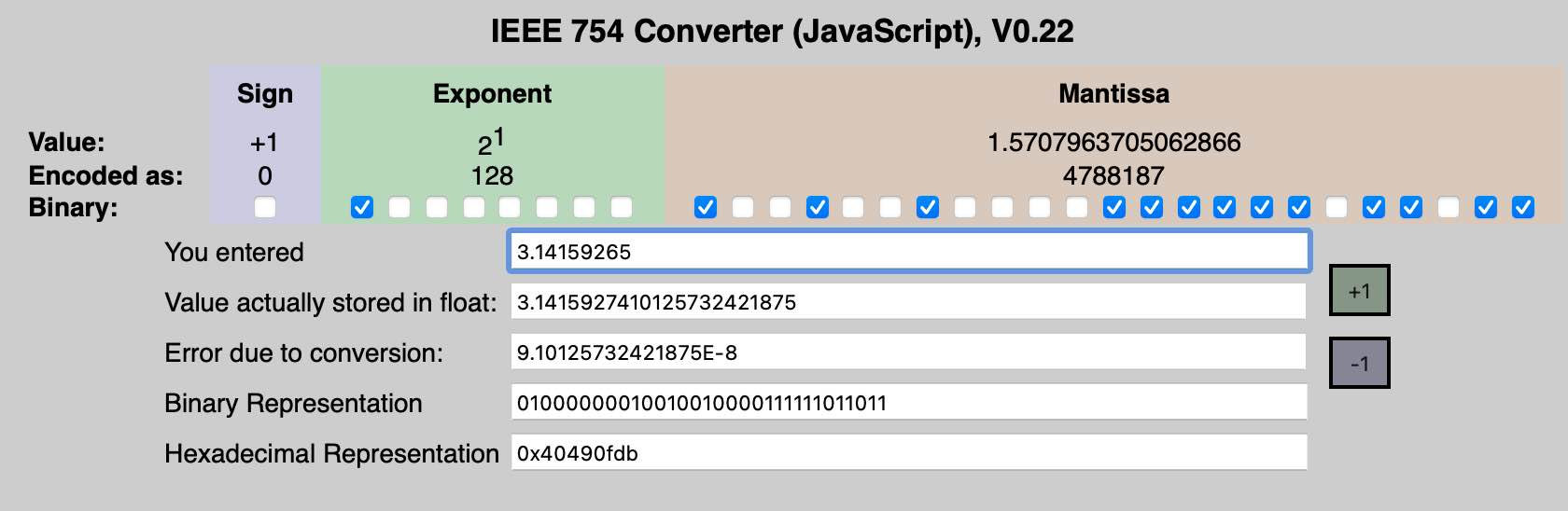The purpose of computing is insight, not numbers.
Richard Hamming（理查德·汉明，美国数学家，在计算机科学和通信工程领域贡献突出，美国计算机协会（ACM）的创立人之一，1968年图灵奖得主）

### 整数平方根

$$\quad$$ 输入: x = 4
$$\quad$$ 输出: 2

$$\quad$$ 输入: x = 8
$$\quad$$ 输出: 2
$$\quad$$ 说明: 8 的平方根是 2.82842..., 由于返回类型是整数，小数部分将被舍去。

#### 二分查找法

• 下界 $$low\leqslant \lfloor\sqrt{x}\rfloor+1$$
• 上界 $$high\geqslant \lfloor\sqrt{x}\rfloor$$

#### 牛顿迭代法1. 第9到16行实现首次估值的设置，这是一个利用比较和移位操作的精巧设计！变量s保存不小于 $$\sqrt a$$ 且值最小的2的幂指数，1 << s$$2^s$$）就是首次估值！
2. 如果存在快速的前导0计数指令或函数nlz()，第9到14行可以用s = 16 - nlz(a - 1)/21代替。
3. 如第17行所示，因为首次估值是2的幂，第一次迭代里的除法用移位代替。
4. 此算法最多执行5次除法，当 $$a\leqslant(2^{24}-1)$$ 时，最多4次。

### 浮点数平方根

#### IEEE 754（来源：英文维基百科条目“IEEE 754”）C语言的float类型对应IEEE 754标准规定的32位单精确度浮点数，double类型对应64位双精确度浮点数。

#### 二分搜索法

• 初始值设定：这里要区分输入参数大于或小于等于1的情况
• 如果输入参数小于1，其平方根比自身要大，所以要将上界设为1，下界为其自身。
• 输入参数小于1的情况正好相反，可将上界设为自身，下界为1。
• 循环结束条件：为避免出现死循环，要注意以下两点
• 由于上下界都为浮点数，必须要定义一个误差范围，比如 $$10^{-6}$$。当上下界差值不超过此值时，立即结束循环。
• 浮点数的精度是有限的。32位浮点数float只可以保证7位十进制有效数字。比如当下界为141.421356、上界为141.421371时，计算出来的中间值还是141.421356。所以还必须加上强制退出的判定。

#### 巴比伦解法

1. 预测一个平方根值 $$x$$（优选接近实际平方根的数值），初始 $$y=a/x$$
2. 计算 $$x=(x+y)/2$$（使用算术平均值近似几何平均值）
3. 比较 $$x$$$$y$$，如果差值达不到精度，重复以上步骤

### 平方根倒数

#### 速算法解析

##### 线性近似### 单元测试

1. 这是因为“nlz”本质上就是以2为底的整数对数函数。对于大于0的整数 $$a$$，有 $$\lceil\log_2(x)\rceil=32-\mathrm{nlz}(x-1)$$，由此导出 $$\lceil\log_2(\sqrt x)\rceil=16-\mathrm{nlz}(x-1)/2$$↩︎

2. James Ulery, Computing Integer Square Roots, University of Toronto, 2006↩︎

3. 耶鲁大学收藏的一块古巴比伦黏土板（编号YBC 7289），上面以六十进制记载了单位正方形对角线长的准确估计值$${\textstyle 1;24,51,10}$$。这个数的估算误差小于两百万分之一。↩︎

4. 注意这种类型转化与C语言的强制类型转换不同，后者会直接舍弃小数位。↩︎

5. Chris Lomont. Fast inverse square root. Technical report, Indiana: Purdue University, 2003.↩︎

6. Moroz, Leonid V., et al. "Fast calculation of inverse square root with the use of magic constant–analytical approach." Applied Mathematics and Computation 316 (2018): 245-255.↩︎